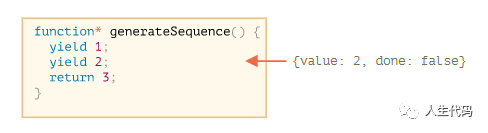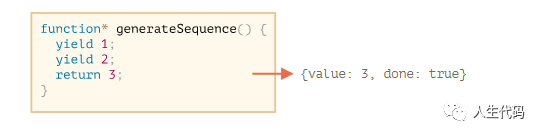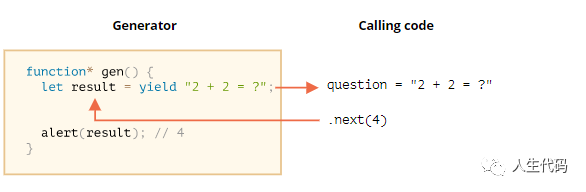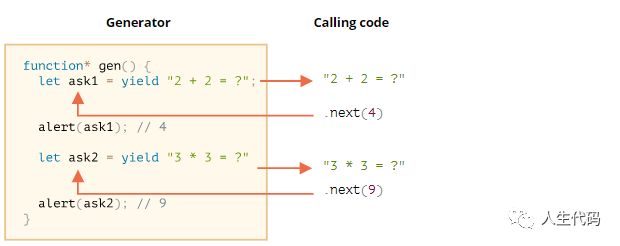2021/03/01 19:46

生成器函数

functiongenerateSequence({
yield 1;
yield 2;
return 3;
}

functiongenerateSequence({
yield 1;
yield 2;
return 3;
}

// "generator function" creates "generator object"
let generator = generateSequence();next()的结果总是一个具有两个属性的对象:

• value:生成的值。
• done:如果函数代码已经完成，则为 true，否则为 false

functiongenerateSequence({
yield 1;
yield 2;
return 3;
}

let generator = generateSequence();

let one = generator.next();

alert(JSON.stringify(one)); // {value: 1, done: false}let two = generator.next();

alert(JSON.stringify(two)); // {value: 2, done: false}generator.next()的新调用不再有意义。如果我们执行它们，它们将返回相同的对象:{done: true}

生成器是可迭代的

functiongenerateSequence({
yield 1;
yield 2;
return 3;
}

let generator = generateSequence();

for(let value of generator) {
}

functiongenerateSequence({
yield 1;
yield 2;
yield 3;
}

let generator = generateSequence();

for(let value of generator) {
alert(value); // 1, then 2, then 3
}

functiongenerateSequence({
yield 1;
yield 2;
yield 3;
}

let sequence = [0, ...generateSequence()];

alert(sequence); // 0, 1, 2, 3

Using generators for iterables

let range = {
from1,
to5,

// for..of range calls this method once in the very beginning
[Symbol.iterator]() {
// ...it returns the iterator object:
// onward, for..of works only with that object, asking it for next values
return {
currentthis.from,
lastthis.to,

// next() is called on each iteration by the for..of loop
next() {
// it should return the value as an object {done:.., value :...}
if (this.current <= this.last) {
return { donefalsevaluethis.current++ };
} else {
return { donetrue };
}
}
};
}
};

// iteration over range returns numbers from range.from to range.to

let range = {
from1,
to5,

*[Symbol.iterator]() { // a shorthand for [Symbol.iterator]: function*()
for(let value = this.from; value <= this.to; value++) {
yield value;
}
}
};

• .next() 方法
• 返回 {value: ..., done: true/false}

生成器组合

functiongenerateSequence(start, end{
for (let i = start; i <= end; i++) yield i;
}

• 首先,数字0...9(字符代码48…57)，
• 后面跟着大写字母A..Z(字符代码65…90)
• 后面跟着小写字母a..z(字符代码97…122)

functiongenerateSequence(start, end{
for (let i = start; i <= end; i++) yield i;
}

// 0..9
yield* generateSequence(4857);

// A..Z
yield* generateSequence(6590);

// a..z
yield* generateSequence(97122);

}

let str = '';

str += String.fromCharCode(code);
}

yield*指令将执行委托给另一个生成器。这个术语意味着yield* gen在生成器生成器上迭代，并透明地将它的yield传递给外部。就好像这些值是由外部生成器生成的一样。

functiongenerateSequence(start, end{
for (let i = start; i <= end; i++) yield i;
}

functiongenerateAlphaNum({

// yield* generateSequence(48, 57);
for (let i = 48; i <= 57; i++) yield i;

// yield* generateSequence(65, 90);
for (let i = 65; i <= 90; i++) yield i;

// yield* generateSequence(97, 122);
for (let i = 97; i <= 122; i++) yield i;

}

let str = '';

for(let code of generateAlphaNum()) {
str += String.fromCharCode(code);
}

yield 是一条双行道

functiongen({
// Pass a question to the outer code and wait for an answer
let result = yield "2 + 2 = ?"// (*)

}

let generator = gen();

let question = generator.next().value; // <-- yield returns the value

generator.next(4); // --> pass the result into the generatorgenerator.next(4)上，生成器恢复，结果为4:let result = 4

// resume the generator after some time
setTimeout(() => generator.next(4), 1000);

functiongen({
let ask1 = yield "2 + 2 = ?";

let ask2 = yield "3 * 3 = ?"

}

let generator = gen();

alert( generator.next().value ); // "2 + 2 = ?"

alert( generator.next(4).value ); // "3 * 3 = ?"• 第一个.next()开始执行…它到达第一个yield。

• 第二个.next(4)将4作为第一个yield的结果传递回生成器，并继续执行。

• 它达到了第二个收益，它成为生成器调用的结果。

• 第三个next(9)将9作为第二个yield的结果传递给生成器，并继续执行到达函数末尾的操作，完成:true。

generator.throw

functiongen({
try {
let result = yield "2 + 2 = ?"// (1)

alert("The execution does not reach here, because the exception is thrown above");
} catch(e) {
}
}

let generator = gen();

let question = generator.next().value;

generator.throw(new Error("The answer is not found in my database")); // (2)

382/5000 在第(2)行抛出到生成器中的错误会导致第(1)行中出现yield异常。在上面的例子中，try..catch抓住它并展示它。

functiongenerate({
let result = yield "2 + 2 = ?"// Error in this line
}

let generator = generate();

let question = generator.next().value;

try {
generator.throw(new Error("The answer is not found in my database"));
catch(e) {
}

总结

• 生成器是由生成器函数function* f(…){…}创建的。

• 生成器(仅)内部存在yield操作符。

• 外部代码和生成器可以通过next/yield调用交换结果。

0
0 收藏

作者的其它热门文章0 评论
0 收藏
0